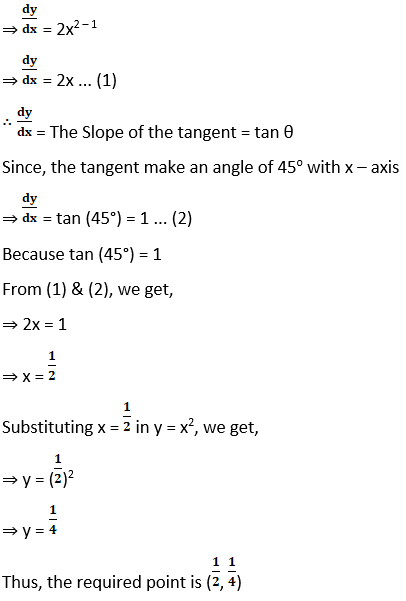# RD Sharma Solutions For Class 12 Maths Exercise 16.1 Chapter 16 Tangents and Normals

RD Sharma Solutions for Class 12 Maths Exercise 16.1 Chapter 16 Tangents and Normals are provided here. This exercise has problems which are solved on slopes of tangents and normals by the subject experts at BYJU’S. Our team of faculty mainly works with the aim of making Mathematics easier for students, based on their grasping power.

Solving the exercise problems will become easier if students first solve the examples, which are present before each exercise. The PDF of RD Sharma Solutions for Class 12 Maths Chapter 16 Tangents and Normals Exercise 16.1 are provided here. Learners of Class 12 can easily download this PDF. Some of the important topics of this exercise are listed below.

• The slope of a line
• Slopes of tangent and normal
• Finding the slopes of the tangent and the normal at a given point
• Finding the point on a given curve at which tangent is parallel or perpendicular to a given line

## RD Sharma Solutions Class 12 Tangents and Normals Exercise 16.1: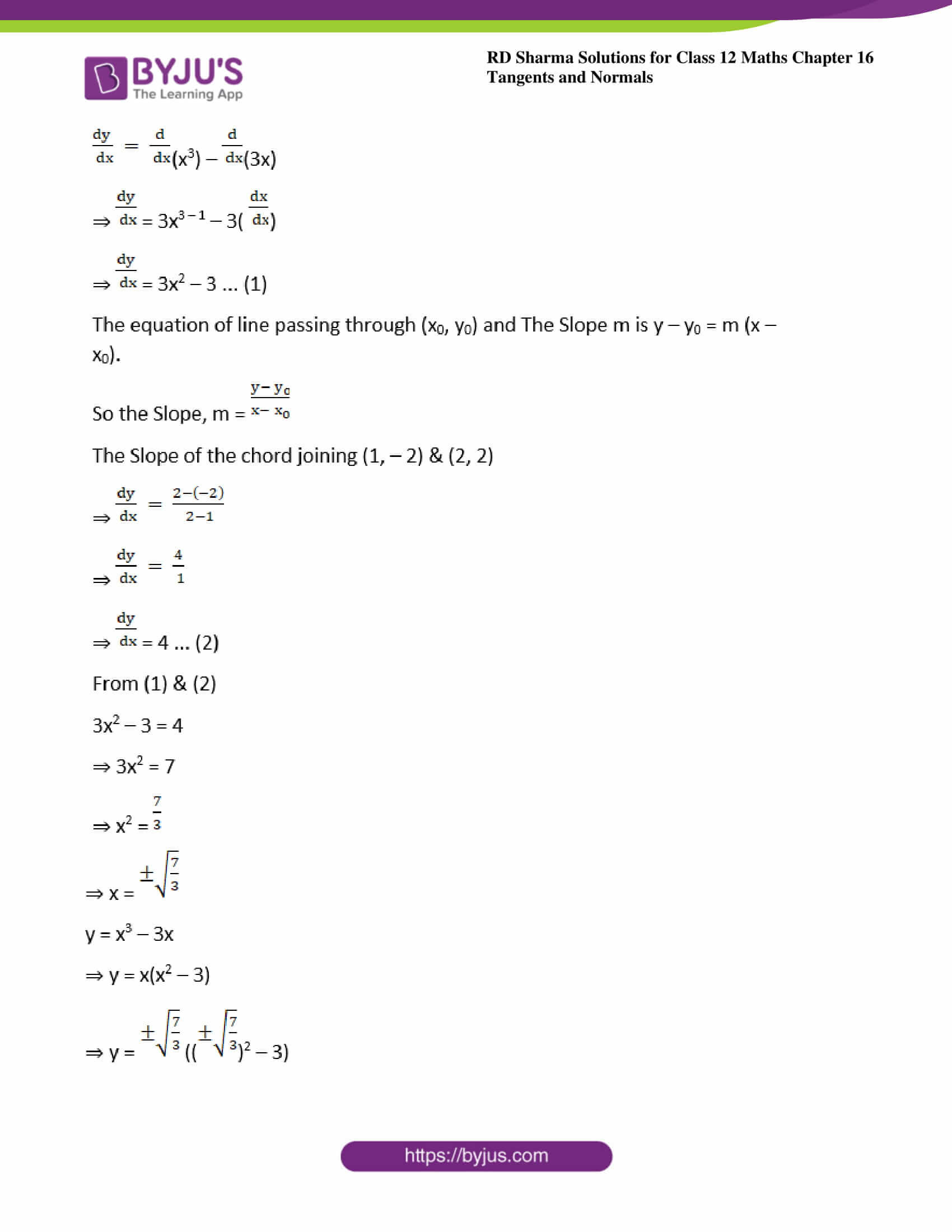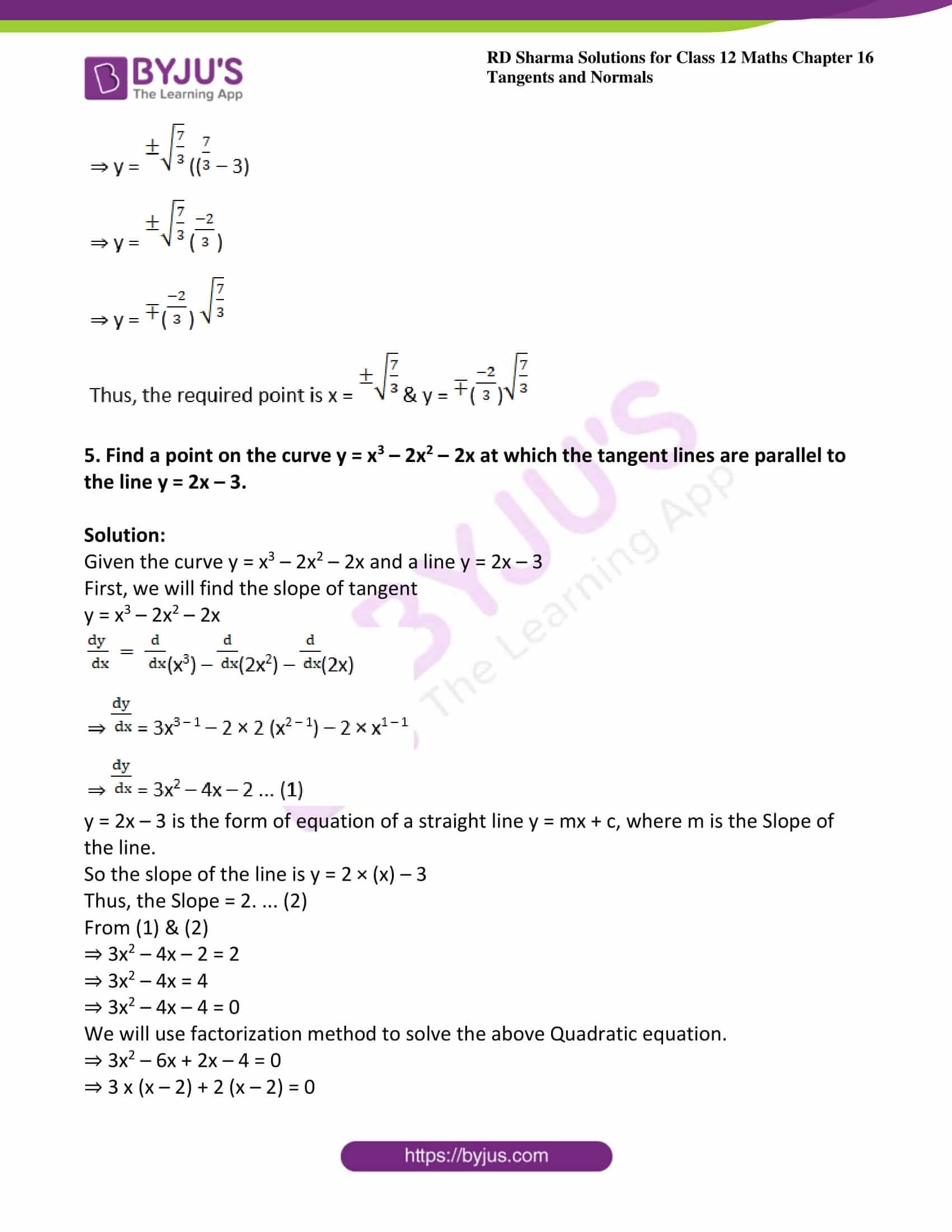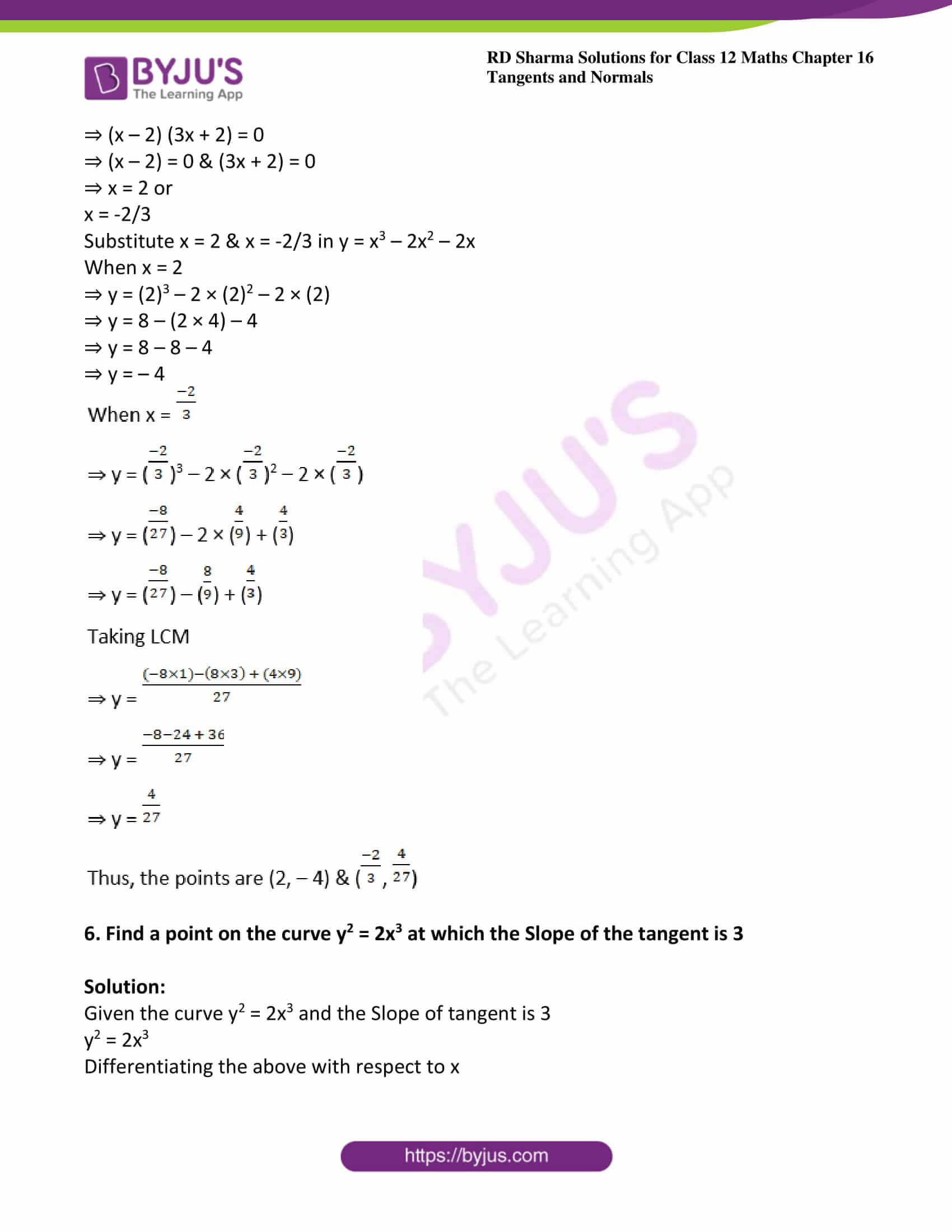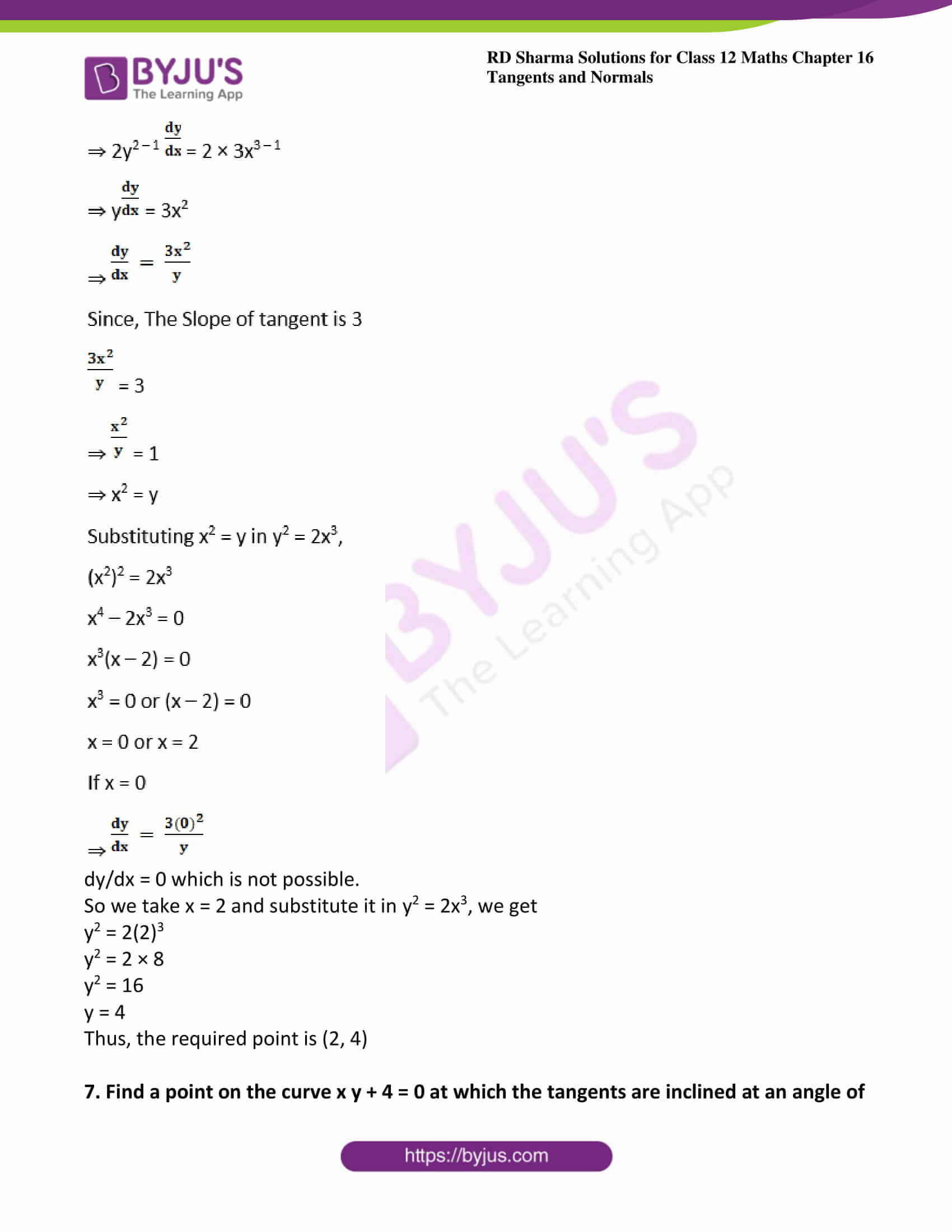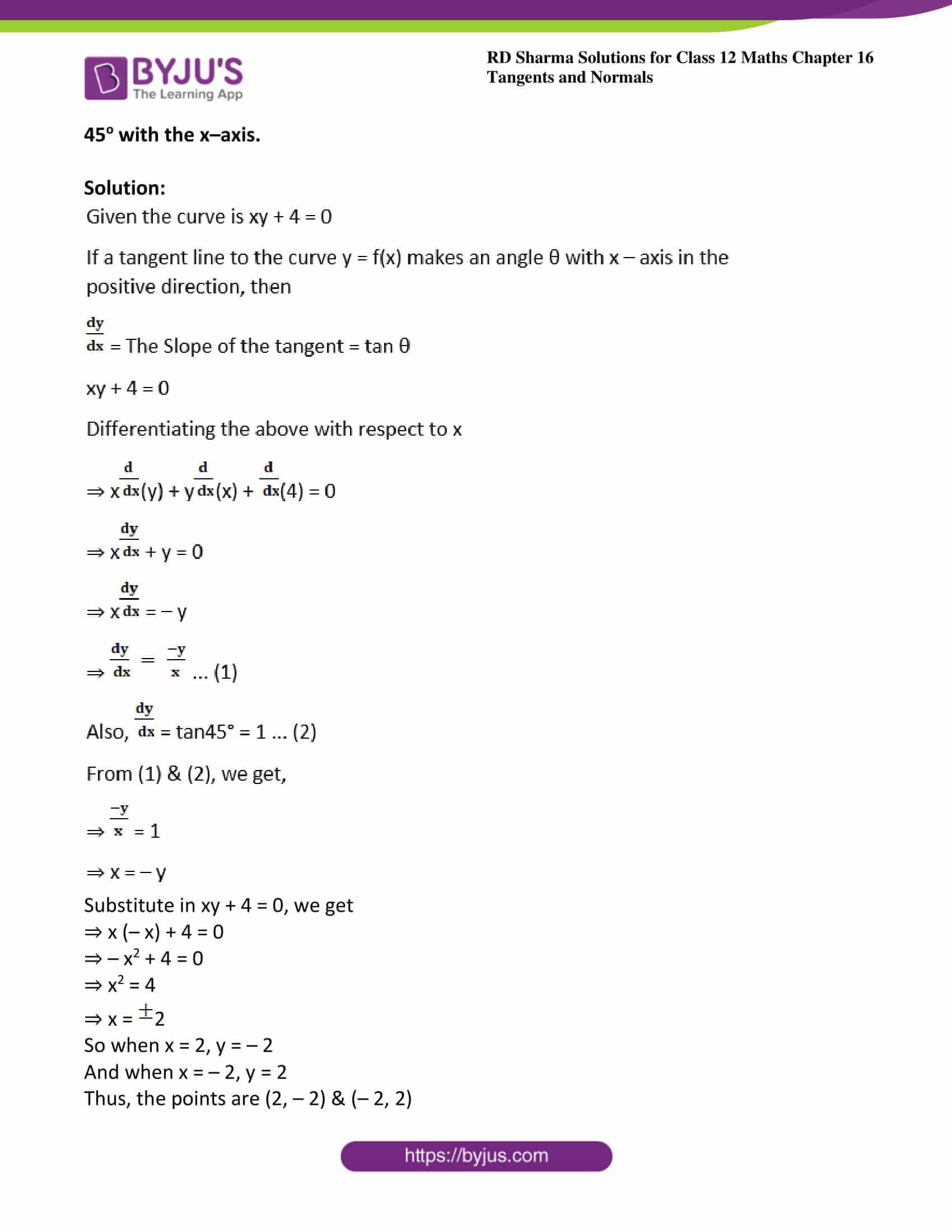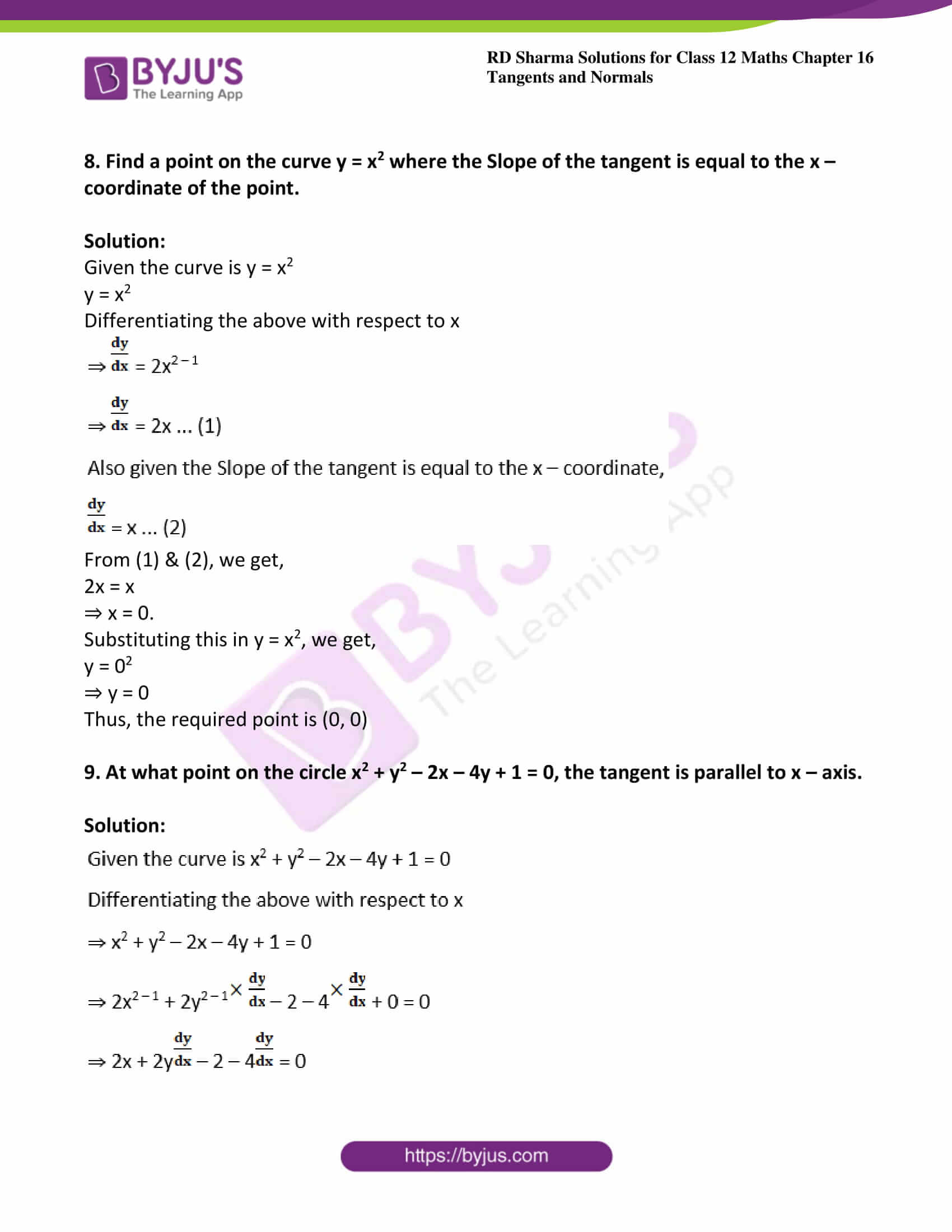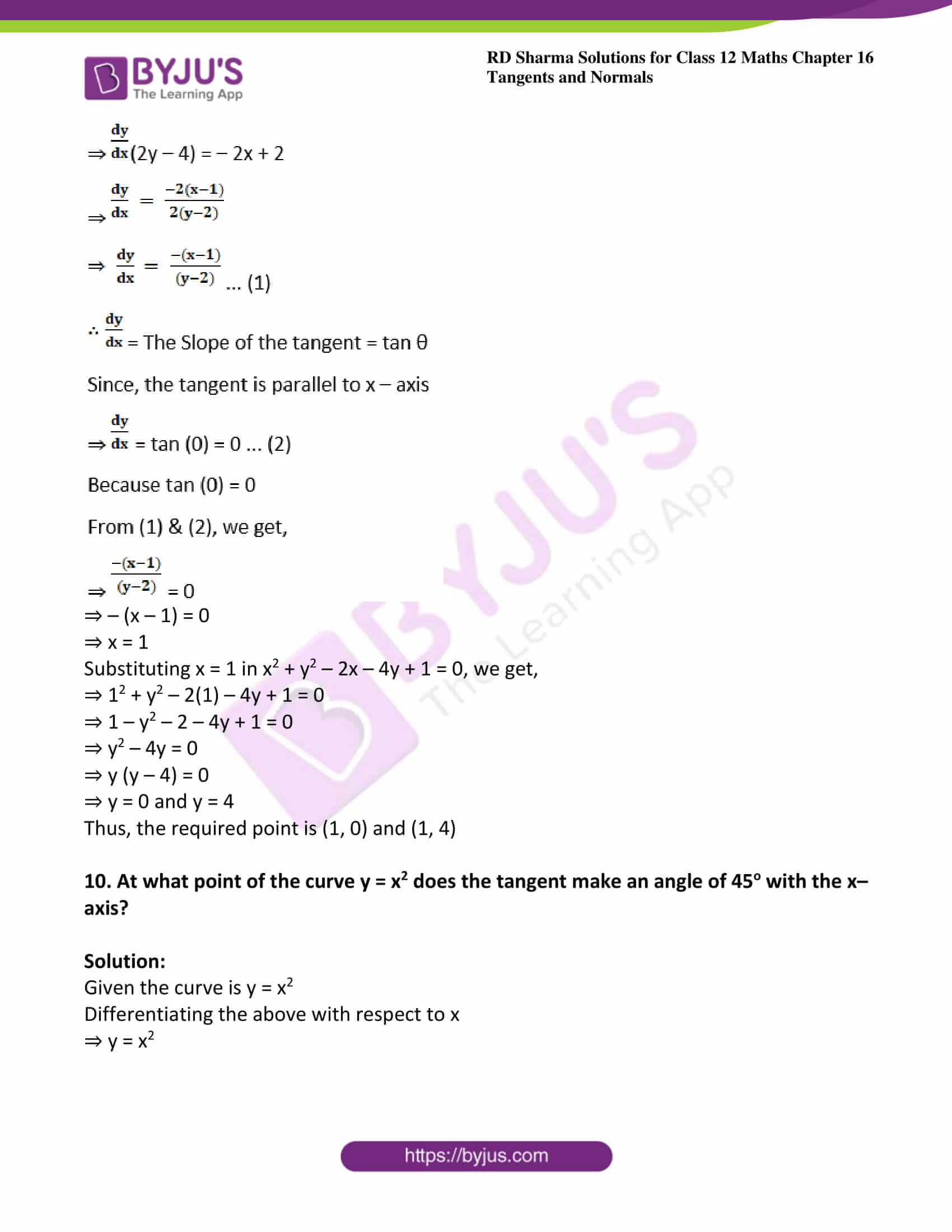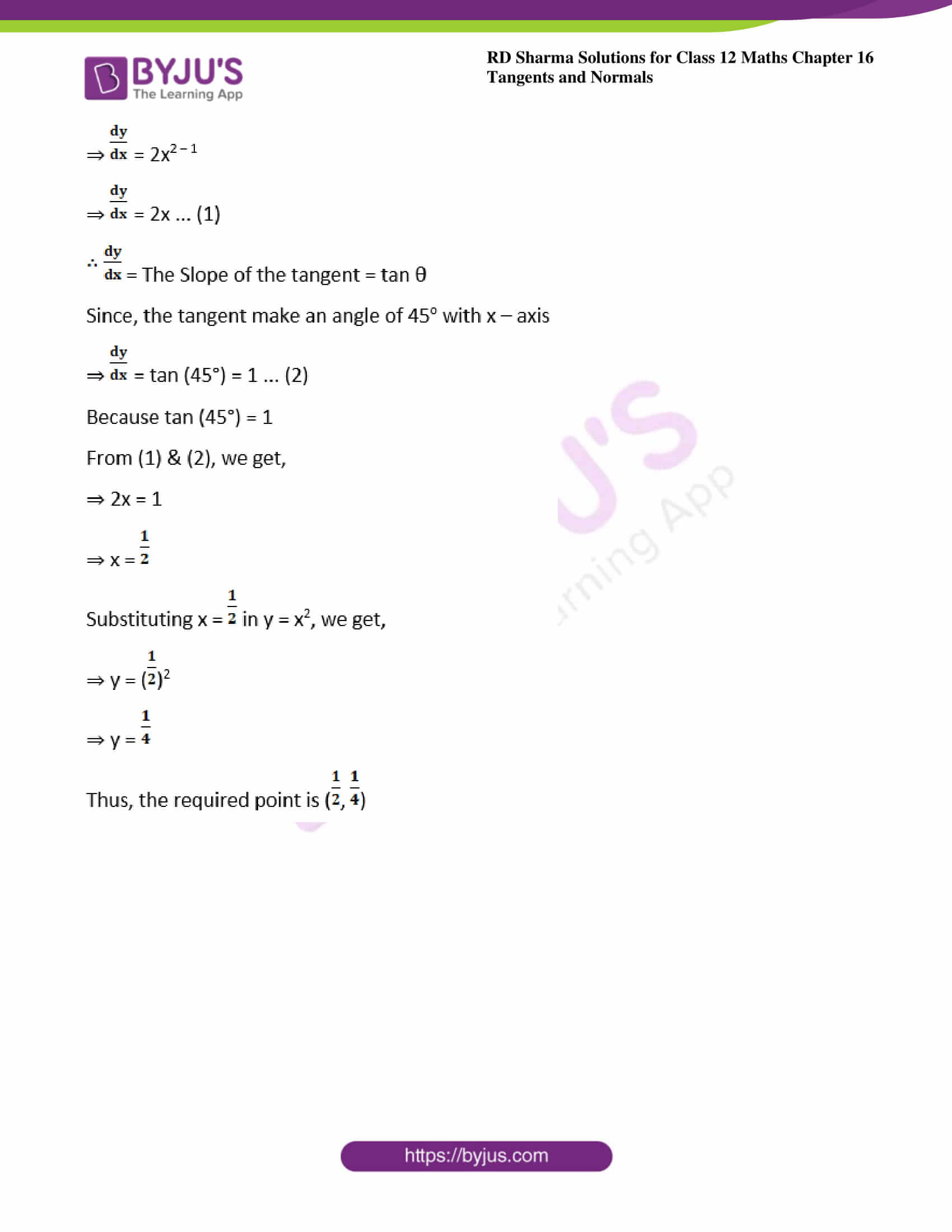### Access other exercises of RD Sharma Solutions For Class 12 Chapter 16 – Tangents and Normals

Exercise 16.2 Solutions

Exercise 16.3 Solutions

### Access answers to Maths RD Sharma Solutions For Class 12 Chapter 15 – Mean Value Theorems Exercise 16.1

Exercise 16.1 Page No: 16.10

1. Find the Slopes of the tangent and the normal to the following curves at the indicated points: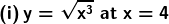Solution: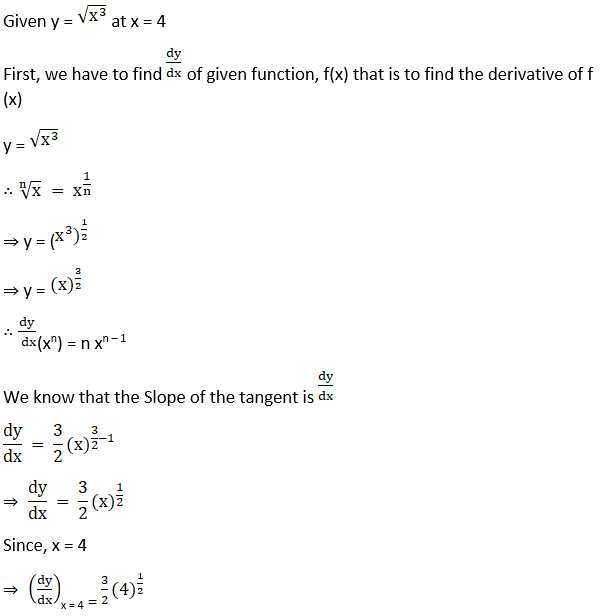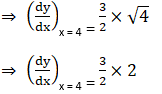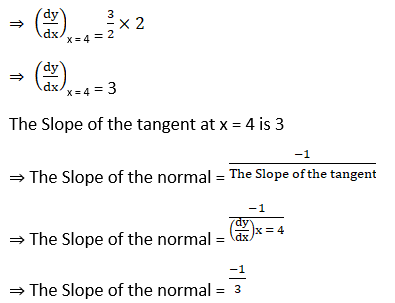(ii) y = √x at x = 9

Solution: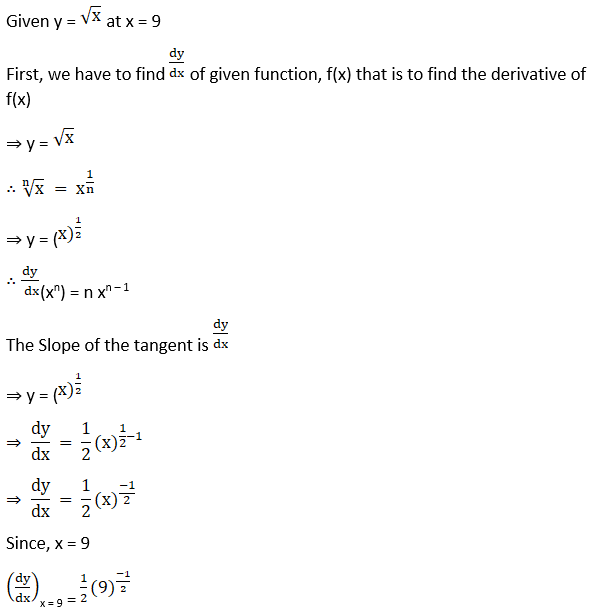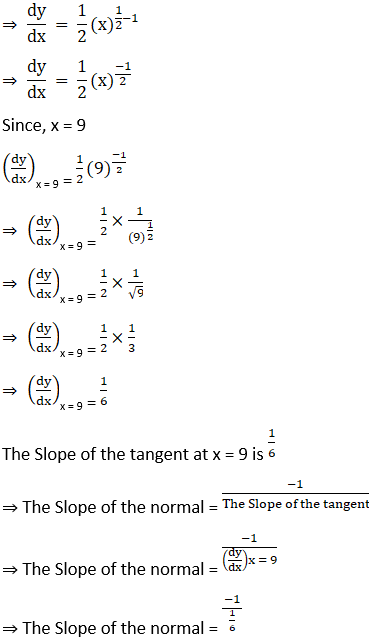⇒ The Slope of the normal = – 6

(iii) y = x3 – x at x = 2

Solution: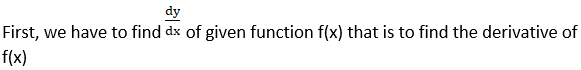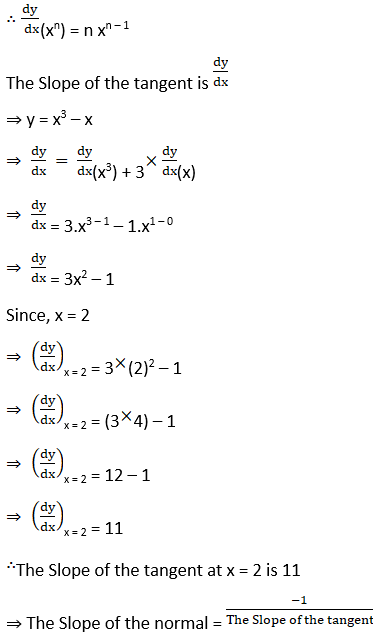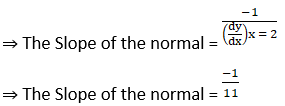(iv) y = 2x2 + 3 sin x at x = 0

Solution: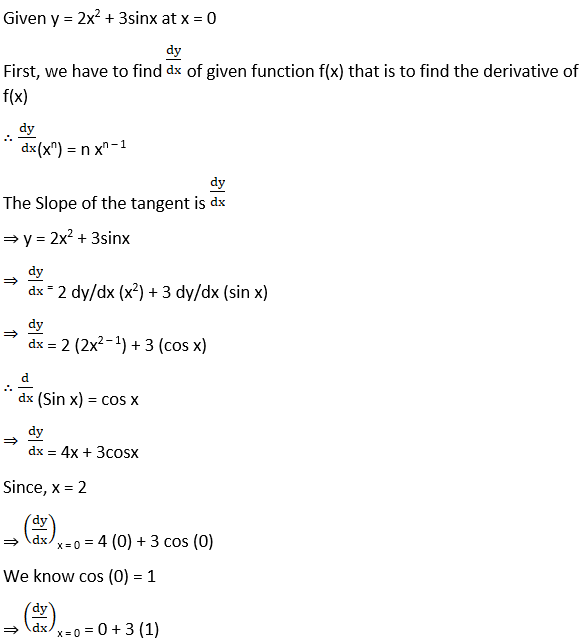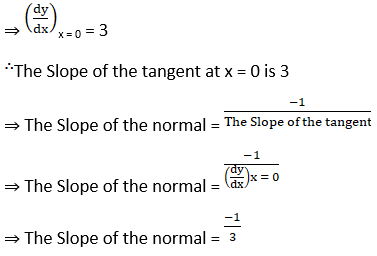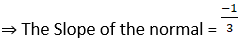(v) x = a (θ – sin θ), y = a (1 + cos θ) at θ = -π /2

Solution: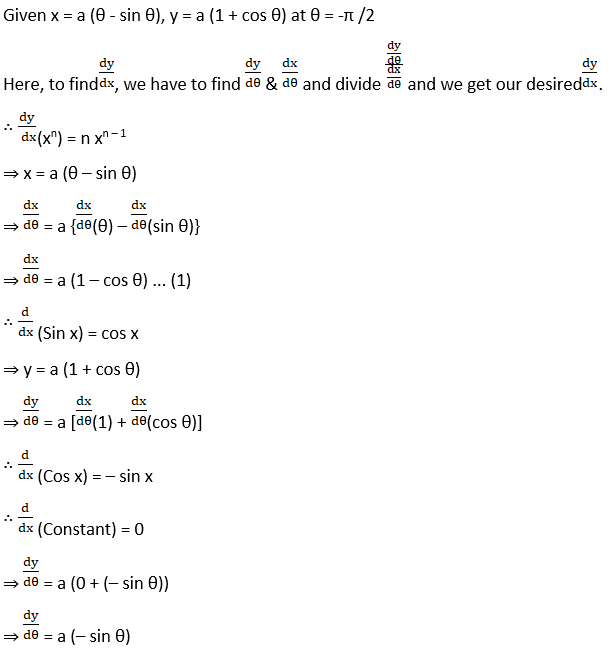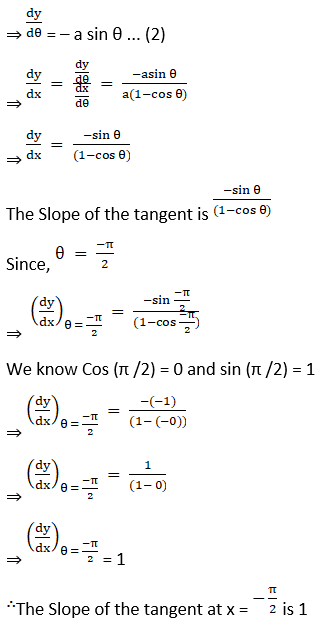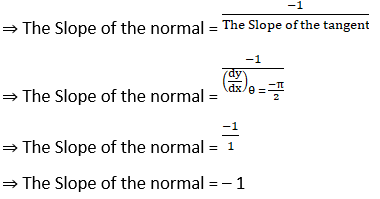(vi) x = a cos3 θ, y = a sin3 θ at θ = π /4

Solution: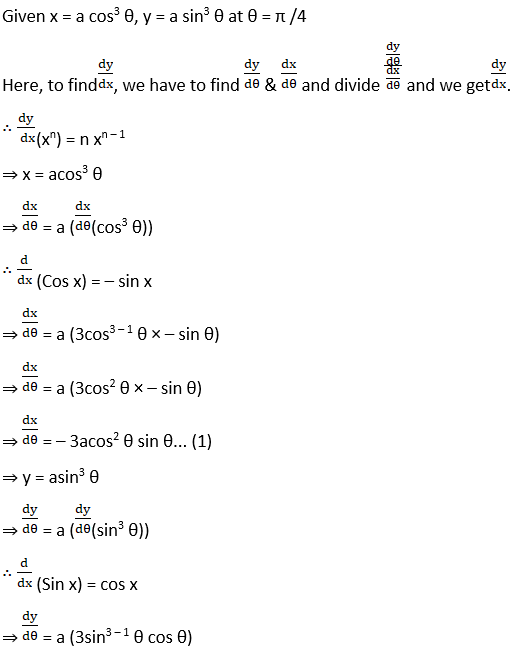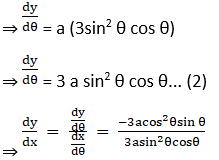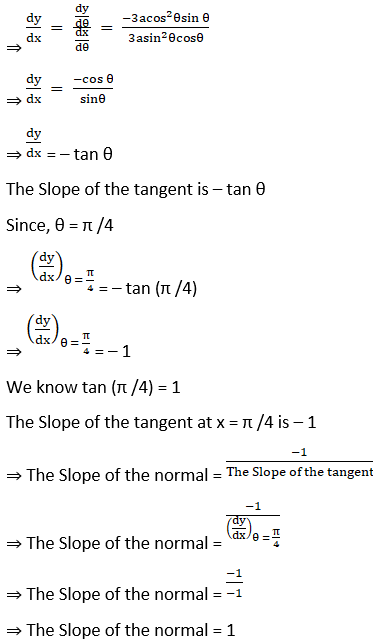(vii) x = a (θ – sin θ), y = a (1 – cos θ) at θ = π /2

Solution: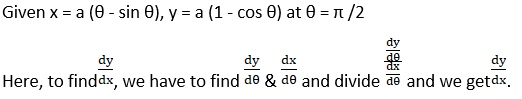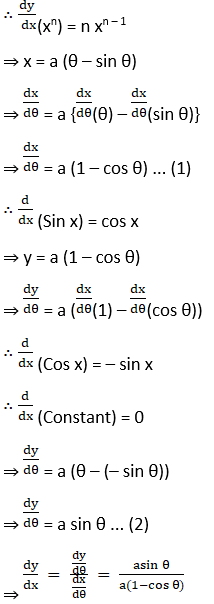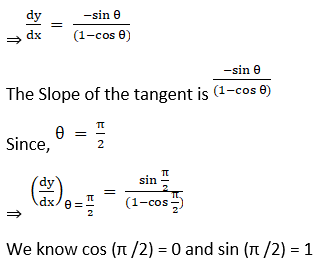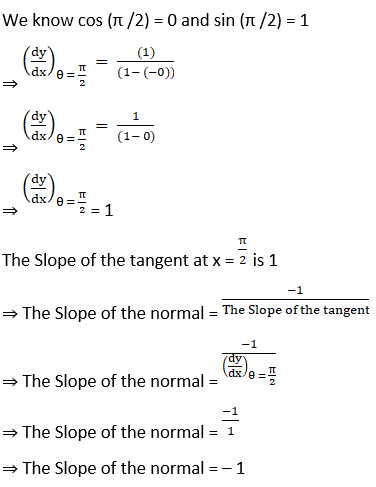(viii) y = (sin 2x + cot x + 2)2 at x = π /2

Solution: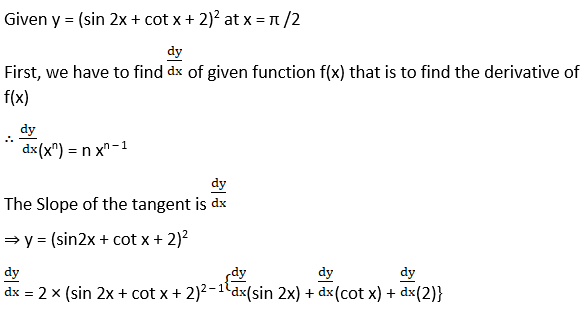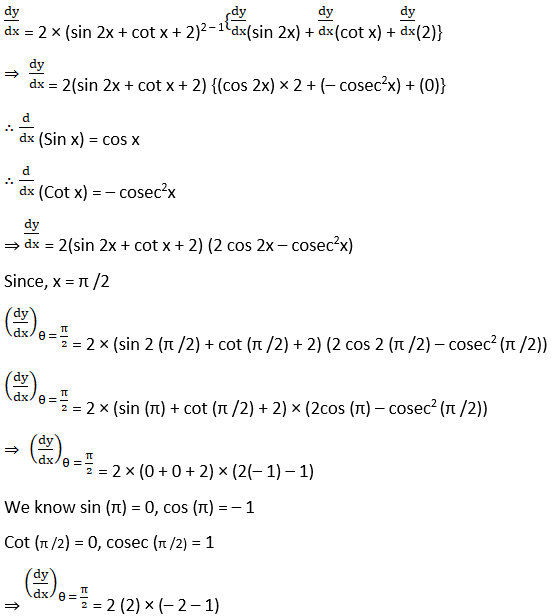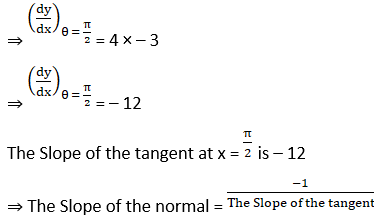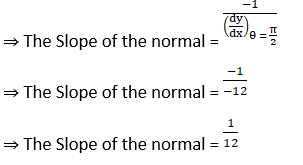(ix) x2 + 3y + y2 = 5 at (1, 1)

Solution: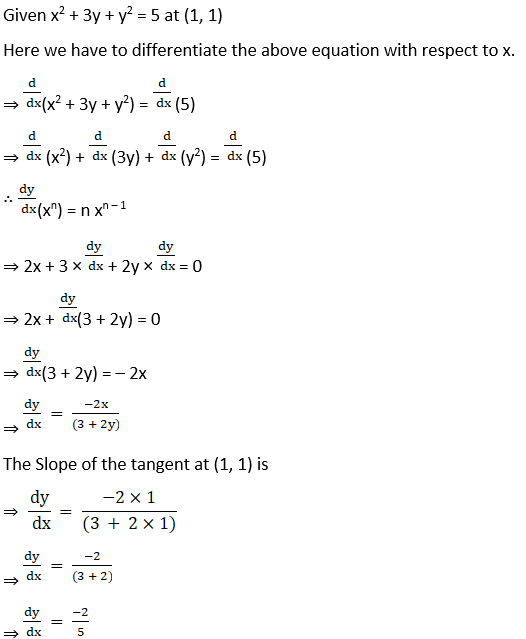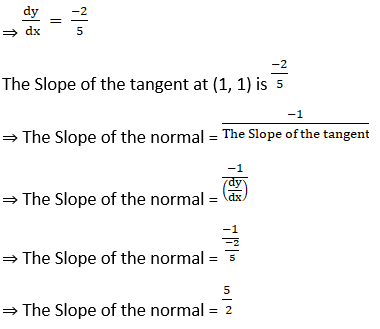(x) x y = 6 at (1, 6)

Solution: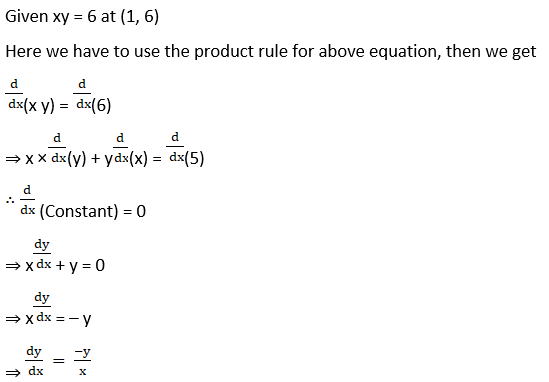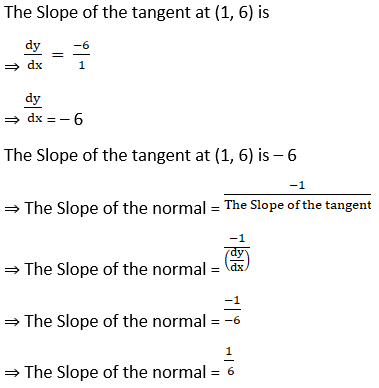2. Find the values of a and b if the Slope of the tangent to the curve x y + a x + by = 2 at (1, 1) is 2.

Solution: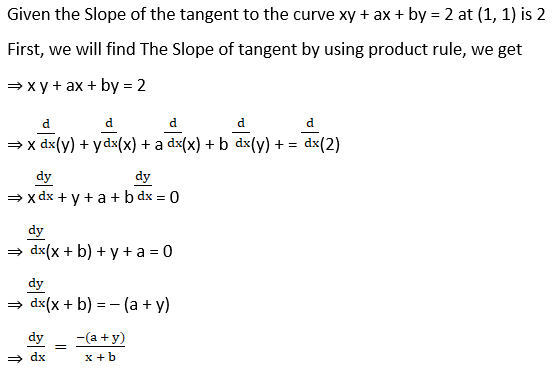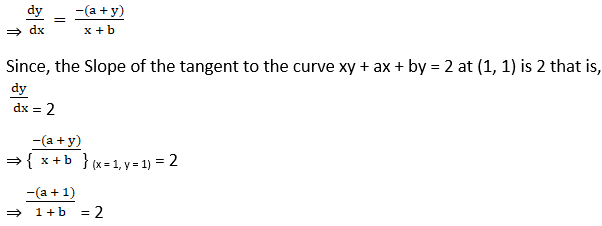⇒ – a – 1 = 2(1 + b)

⇒ – a – 1 = 2 + 2b

⇒ a + 2b = – 3 … (1)

Also, the point (1, 1) lies on the curve xy + ax + by = 2, we have

1 × 1 + a × 1 + b × 1 = 2

⇒ 1 + a + b = 2

⇒ a + b = 1 … (2)

From (1) & (2), we get b = -4

Substitute b = – 4 in a + b = 1

a – 4 = 1

⇒ a = 5

So the value of a = 5 & b = – 4

3. If the tangent to the curve y = x3 + a x + b at (1, – 6) is parallel to the line x – y + 5 = 0, find a and b

Solution: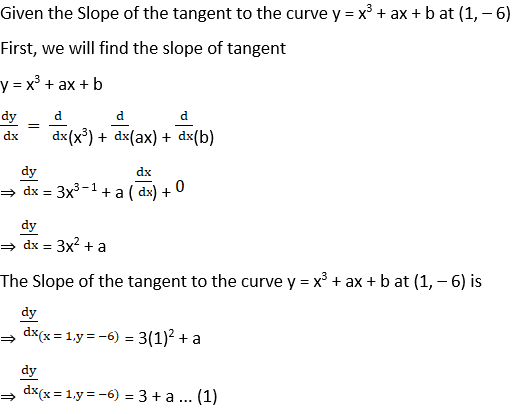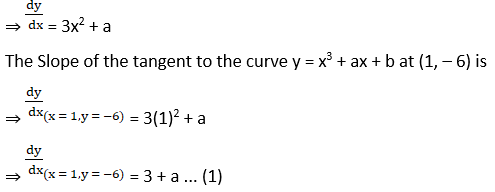The given line is x – y + 5 = 0

y = x + 5 is the form of equation of a straight line y = mx + c, where m is the Slope of the line.

So the slope of the line is y = 1 × x + 5

So the Slope is 1. … (2)

Also the point (1, – 6) lie on the tangent, so

x = 1 & y = – 6 satisfies the equation, y = x3 + ax + b

– 6 = 13 + a × 1 + b

⇒ – 6 = 1 + a + b

⇒ a + b = – 7 … (3)

Since, the tangent is parallel to the line, from (1) & (2)

Hence, 3 + a = 1

⇒ a = – 2

From (3)

a + b = – 7

⇒ – 2 + b = – 7

⇒ b = – 5

So the value is a = – 2 & b = – 5

4. Find a point on the curve y = x3 – 3x where the tangent is parallel to the chord joining (1, – 2) and (2, 2).

Solution: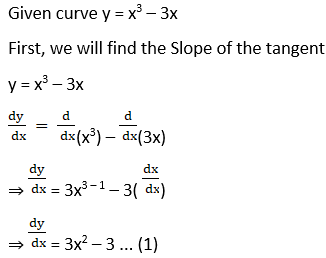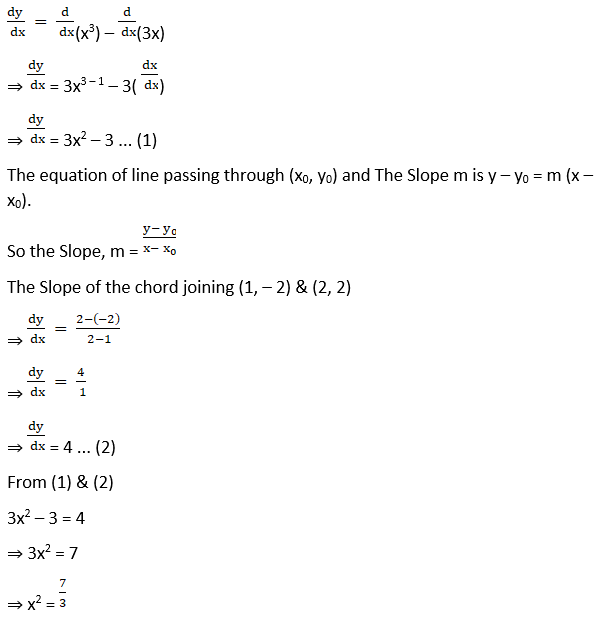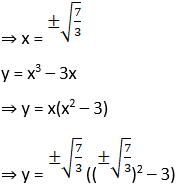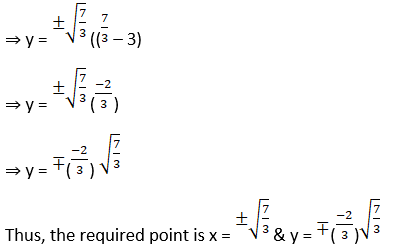5. Find a point on the curve y = x3 – 2x2 – 2x at which the tangent lines are parallel to the line y = 2x – 3.

Solution:

Given the curve y = x3 – 2x2 – 2x and a line y = 2x – 3

First, we will find the slope of tangent

y = x3 – 2x2 – 2x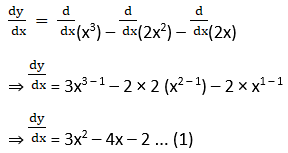y = 2x – 3 is the form of equation of a straight line y = mx + c, where m is the Slope of the line.

So the slope of the line is y = 2 × (x) – 3

Thus, the Slope = 2. … (2)

From (1) & (2)

⇒ 3x2 – 4x – 2 = 2

⇒ 3x2 – 4x = 4

⇒ 3x2 – 4x – 4 = 0

We will use factorization method to solve the above Quadratic equation.

⇒ 3x2 – 6x + 2x – 4 = 0

⇒ 3 x (x – 2) + 2 (x – 2) = 0

⇒ (x – 2) (3x + 2) = 0

⇒ (x – 2) = 0 & (3x + 2) = 0

⇒ x = 2 or

x = -2/3

Substitute x = 2 & x = -2/3 in y = x3 – 2x2 – 2x

When x = 2

⇒ y = (2)3 – 2 × (2)2 – 2 × (2)

⇒ y = 8 – (2 × 4) – 4

⇒ y = 8 – 8 – 4

⇒ y = – 4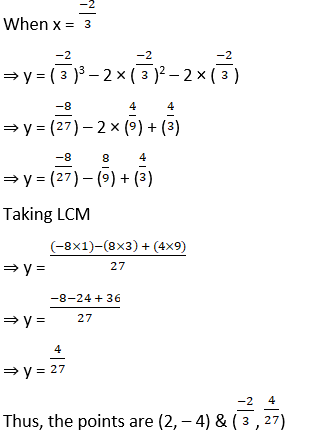6. Find a point on the curve y2 = 2x3 at which the Slope of the tangent is 3

Solution:

Given the curve y2 = 2x3 and the Slope of tangent is 3

y2 = 2x3

Differentiating the above with respect to x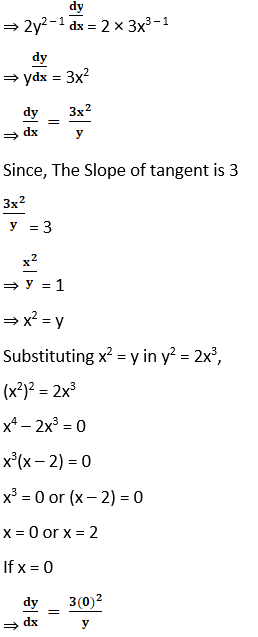dy/dx = 0 which is not possible.

So we take x = 2 and substitute it in y2 = 2x3, we get

y2 = 2(2)3

y2 = 2 × 8

y2 = 16

y = 4

Thus, the required point is (2, 4)

7. Find a point on the curve x y + 4 = 0 at which the tangents are inclined at an angle of 45o with the x–axis.

Solution: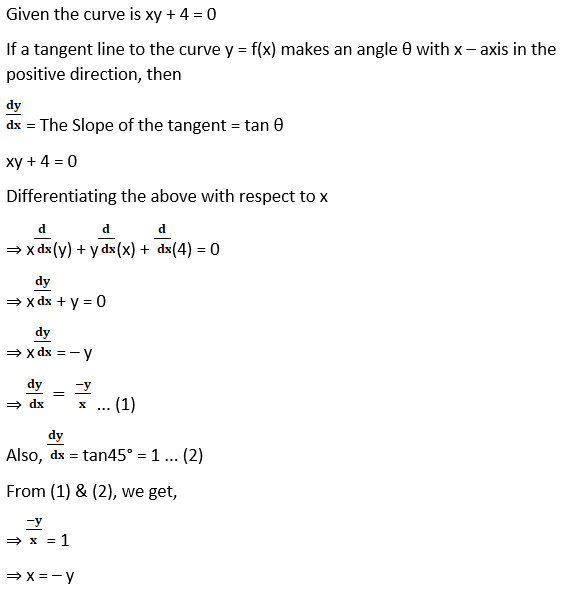Substitute in xy + 4 = 0, we get

⇒ x (– x) + 4 = 0

⇒ – x2 + 4 = 0

⇒ x2 = 4

⇒ x =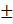2

So when x = 2, y = – 2

And when x = – 2, y = 2

Thus, the points are (2, – 2) & (– 2, 2)

8. Find a point on the curve y = x2 where the Slope of the tangent is equal to the x – coordinate of the point.

Solution:

Given the curve is y = x2

y = x2

Differentiating the above with respect to x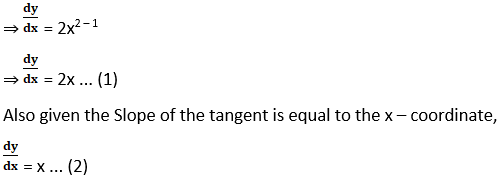From (1) & (2), we get,

2x = x

⇒ x = 0.

Substituting this in y = x2, we get,

y = 02

⇒ y = 0

Thus, the required point is (0, 0)

9. At what point on the circle x2 + y2 – 2x – 4y + 1 = 0, the tangent is parallel to x – axis.

Solution: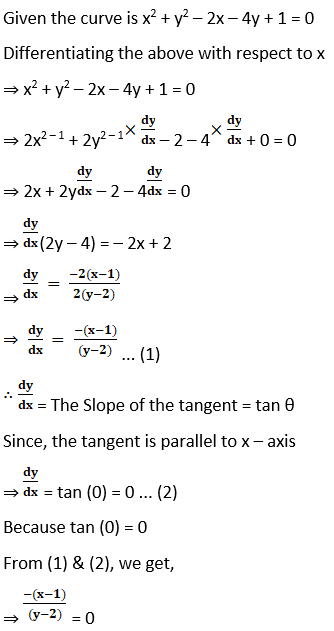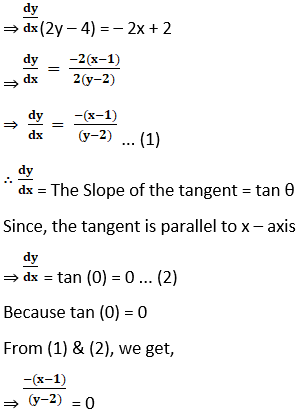⇒ – (x – 1) = 0

⇒ x = 1

Substituting x = 1 in x2 + y2 – 2x – 4y + 1 = 0, we get,

⇒ 12 + y2 – 2(1) – 4y + 1 = 0

⇒ 1 – y2 – 2 – 4y + 1 = 0

⇒ y2 – 4y = 0

⇒ y (y – 4) = 0

⇒ y = 0 and y = 4

Thus, the required point is (1, 0) and (1, 4)

10. At what point of the curve y = x2 does the tangent make an angle of 45o with the x–axis?

Solution:

Given the curve is y = x2

Differentiating the above with respect to x

⇒ y = x2